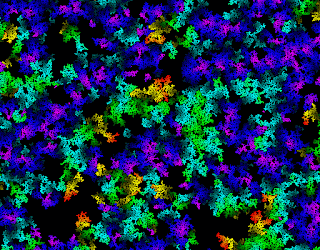Thursday, January 08, 2009

Cosmogenic drift and stochastic processesI recently proposed an extension to Lee Smolin's theory of cosmological natural selection, in which I attempt to explain the evolution of a quantum relativistic universe, a necessary precondition for a population of universes evolving by natural selection. I propose that the parameters of physics not only change when a new universe is born, but undergo random diffusion even in universes which cannot initially reproduce. I refer to this process as cosmogenic drift, by analogy with the process of genetic drift in evolutionary biology.

Random diffusion is a type of stochastic process, so if the theory of cosmogenic drift is to be developed, and if observable predictions are to be derived from it, then it will be necessary to employ the mathematics of stochastic processes. Random diffusion comes in a number of different varieties, so the first question one might pose is to ask which particular type is being suggested here. The simplest type of diffusion is Brownian motion, (also termed a Wiener process), which is a simple random walk in which the increments between random variables St have a normal distribution with a mean value of zero. Geometric Brownian motion, in contrast, is such that each random variable St has a lognormal distribution. Moreover, Brownian motion and geometric Brownian motion can possess a drift, which ensures that the mean values of the random variables St evolve as if under the action of an external force. For example, the Black-Scholes equation, used to calculate the price of financial options, assumes that the value of underlying stocks will evolve according to geometric Brownian motion with drift. This stochastic process is typically denoted as

dSt = μ Stdt + σ St dWt ,

where μ is the percentage drift due to the expected risk-free rate-of-return on the underlying stock, μ St is the drift-rate, σ is the volatility of the stock, σ St is the diffusion rate, and dWt is a standard Wiener process.

In terms of the probability distribution ρ on the range of the random variables St, in the case of simple Brownian motion it evolves according to the diffusion equation,

∂ ρ/∂ t = D ∇2 ρ ,

whilst in the case of Brownian motion with drift tending towards a terminal drift-rate ν, it will evolve according to the diffusion equation with drift:

∂ ρ/∂ t = D ∇2 ρ - ν ∇ ρ .

D here is the so-called diffusion coefficient, ∇2 is the Laplacian, and ∇ is the gradient.

To explain the prior evolution of a quantum relativistic universe, diffusion seems to work equally well as diffusion-with-drift. Simple random diffusion will eventually evolve a universe into a quantum relativistic universe, at which point a population of reproducing universes can be created, and evolution by natural selection can kick-in. Diffusion-with-drift towards the relevant part of the cosmic fitness landscape will produce a quantum relativistic universe in shorter time, but given eternity, this seems unnecessary. Similar considerations suggest that the form of the stochastic process distribution, whether it is normal, lognormal, or otherwise, is superfluous. All of which seems to mitigate against the possibility of deriving potentially observable predictions from the theory. It might still be possible to observe the variations in the parameters of physics within our own universe, and to infer the nature of the stochastic process, but that information would then have to be fed back into the theory, rather than derived from it.